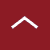# Down to the last detail!Flow simulation

## CFD flow simulation: a sound technology for calculating natural laws.

Analytical basis
Anyone standing on a riverbank and looking at the river immediately notices the complex movements of the flow. Although flow processes can be described analytically for more than 200 years by the partial differential equations of flight dynamics by Navier and Stokes, they could not be solved until now because they are highly non-linear.

Calculation method
It was only in the course of the development of modern computer technologies that flow simulation emerged, which is based on the numerical solution of the Navier-Stokes equations. Computational Fluid Dynamics, CFD, makes the infinite degrees of freedom of the fluid continuum calculable by means of suitable mathematical procedures. The flow equations can additionally be coupled with energy and mass transport equations in order to take heat transport and concentration distributions into account. This means that CFD simulation can be used to calculate both flow and heat processes in detail and make them accessible for engineering.

Discretisation makes it calculable
This requires discretisation, i.e. a reduction of the continuum to a finite number of points. To do this, the space is divided into many small volumes (elements). This is done with the help of computational fluid dynamics software programmes and results in a computational mesh. For flow simulations, high-quality meshes consist of hexahedron or polyhedron elements. With the help of these elements, the physical quantities calculated on the nodes are extrapolated to the entire element volume. Established methods for such an approach are
finite element method (FEM) and finite volume method (FVM).

Simulation model
A simulation model is created on the basis of the computational mesh by adding the relevant physical models (turbulent, laminar, multiphase, etc.), the material parameters (density, viscosity, thermal conductivity, etc.) and the boundary conditions (volume flow, temperature, static friction, etc.). The selection of high-quality CFD software tools is important here, as this is the only way to ensure the implementation of the necessary physical models and the high numerical accuracy.

Simulation result
The numerical flow calculation then provides the desired physical quantities at every point in space. Velocity and temperature distributions, pressures, streamlines, etc. can be visually mapped and quantitatively and qualitatively evaluated. The quality of the simulation model, the calculation and thus also the reliability of the results depend very much on the correct correlation of the computational mesh and the physics to be solved. Both the correct modelling and the meaningful analysis and interpretation of the simulation results require sound experience.

## The difference:

Development of Finite Element Method (FEM)
The first successful method to develop was the finite element method (FEM), which has its roots (1950-60s) in civil engineering and arose from the numerical calculation of trusses and supporting structures. When it was possible to describe the physics of beams numerically (beam elements), the basic principle of the FEM was born: the description of the space between the connection points (nodes). A little later, this method was extended to 2D elements (shells) and 3D elements. The FEM is therefore the 'natural' formulation in structural mechanics, but there are also Computational Fluid Dynamics codes based on the FEM. Commercial FEM programs suitable for industrial use have been available since the 1970s.

Development of Finite Volume Method (FVM)
The development in fluid dynamics was somewhat different. There are no natural connection points in space like in load-bearing structures. Flows are always extended throughout space and have no internal structure. The physical equations are also non-linear in principle and therefore much more difficult to solve than in structural mechanics (e.g. turbulence). In this case, the differential equations are also discretised by decomposing the space into small volumes.

The basic idea here is that everything that flows out of a small volume must flow into the surrounding volumes. This is how the finite volume method (FVM) came about, which is the 'natural' method for fluid dynamics. Since the matrix equations to be solved are (were) still very extensive and only became solvable in the course of increasingly powerful computers, the development of CFD has progressed with a significant time lag compared to structural mechanics. Commercial CFD programmes suitable for industrial use have therefore only been available since around the mid-1980s.Optimierte Form für höchste Ausdauer

Rekordhalter im Langstrecken fliegen ist die Küstenseeschwalbe: Der Vogel brütet in der Arktis und fliegt zum Überwintern in die Antarktis. Dabei legt die Küstenseeschwalbe hin und zurück zwischen 30.000 und 50.000 Kilometer zurück. Das entspricht dem Umfang der Erde. Diese extreme Leistung gelingt der Küstenseeschwalbe nur durch ihren optimierten Körper- und Flügelbau.

Diese Optimierung ist über Millionen von Jahren entstanden – wenn Sie nicht soviel Zeit haben, nutzen Sie einfach den fluio Entwicklungsprozess für F&E!
x